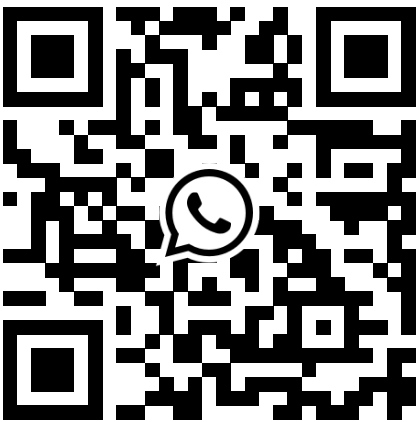﻿ Things You Don't Know About the Din Energy Meter - Acrel Co., Ltd.
English

# Things You Don't Know About the Din Energy Meter

Nowadays, maybe you don't have a wristwatch, but you definitely have a din energy meter at home. As a measuring instrument related to people's livelihood, the din energy meter is a necessary tool for measuring electricity consumption and settling electricity bills. Do you have any questions about the din energy meter? Next, we will summarize some common issues about the din energy meter in general.

## From the appearance of the din energy meter, what kind of din energy meter meets the legal requirements?

The nameplate on the din energy meter should contain at least the following information: din energy meter name, model, factory number, accuracy level, din energy meter constant, reference frequency, reference voltage, reference current and maximum current, the product standard code, manufacturing plant name or trademark, production date, measurement unit, number of decimal places or indication multiplier for the measuring instrument. In addition, qualified din energy meters should have the following identification:

• The nameplate of the din energy meter should have the symbol of the National Metrology Manufacturing Permit (CMC) and the permit number;

• The outer casing of the din energy meter should have the calibration certificate of the calibration institution and the valid period should be marked.

## Why is there a red light flashing when using the din energy meter?

Each din energy meter has a red indicator light - the pulse light. The pulse indicator light of the din energy meter will flash when using electricity. The pulse constant, such as "1600imp/kWh" marked on the din energy meter nameplate, is the number of pulses per kilowatt-hour, which means that the pulse light flashes 1600 times when the customer consumes 1 kilowatt-hour of electricity. The flashing frequency of the pulse light varies with the size of the power load. The larger the power load, the faster the electricity is consumed, and the faster the pulse light flashes.

## How to understand the current specification of the din energy meter?

The capacity of the din energy meter is expressed in the maximum current. For example, the din energy meter parameter of a certain model is 220V, 5(20)A, which means that the rated voltage of the din energy meter is 220V, the basic current is 5A, and the maximum rated current is 20A. If the load used exceeds the maximum current of the din energy meter, the din energy meter may be burned out, or even cause a fire.

## Why do din energy meters need to be rotated periodically?

As the din energy meter is used for a long time, mechanical wear and electronic component aging will often cause measurement errors to exceed the internationally allowed error limits. To ensure the measurement accuracy of the din energy meter, it needs to be disassembled for periodic verification according to relevant national regulations.

## Is the smart din energy meter faster than the mechanical din energy meter?

Smart din energy meters are more sensitive than mechanical din energy meters. Traditional din energy meters can turn extremely slowly or not at all when using appliances that consume a few watts or even less, but smart din energy meters can accurately measure any small current and make the din energy meter rotate. In addition, traditional din energy meters are usually an "induction-type din energy meter" with a disc that rotates with the amount of electricity used, supported by bearings. As time goes by, the bearings gradually age, friction increases, and the rotation speed will become slower, causing the paid fee to be less than the actual power used. Smart din energy meters do not have this problem. Therefore, replacing the traditional din energy meter with a smart one may give you the feeling that the din energy meter is running faster than before.•